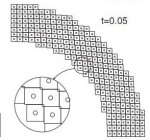# Annulus Twist as a verification test

Illustrated below is the solution to an idealized problem of a linear elastic annulus (blue) subjected to twisting motion caused by rotating the T-bar an angle$\alpha$.  The motion is presumed to be applied slowly enough that equilibrium is satisfied.This simple problem is taken to be governed by the equations of equilibrium$\vec{\nabla}\cdot\sigma=0$, along with the plane strain version of Hooke’s law in which Cauchy stress is taken to be linear with respect to the small strain tensor (symmetric part of the displacement gradient).  If this system of governing equations is implemented in a code, the code will give you an answer, but it is up to you to decide if that answer is a reasonable approximation to reality. This observation helps to illustrate the distinction between verification (i.e., evidence that the equations are solved correctly) and validation (evidence that physically applicable and physically appropriate equations are being solved).  The governing equations always have a correct answer (verification), but that answer might not be very predictive of reality (validation).# Tutorial: Large deformation kinematics

Summarizes the meaning of the deformation gradient tensor, stretches, rotations, etc. Also shows how material line segments, volumes, and area vectors change in response to deformation.

# Publication: On the thermodynamic requirement of elastic stiffness anisotropy in isotropic materials

T. Fuller and R.M. Brannon

In general, thermodynamic admissibility requires isotropic materials develop reversible deformation induced anisotropy (RDIA) in their elastic stiffnesses. Taking the elastic potential for an isotropic material to be a function of the strain invariants, isotropy of the elastic stiffness is possible under distortional loading if and only if the bulk modulus is independent of the strain deviator and the shear modulus is constant. Previous investigations of RDIA have been limited to applications in geomechanics where material non-linearityand large deformations are commonly observed. In the current paper, the degree of RDIA in other materials is investigated. It is found that the resultant anisotropy in materials whose strength does not vary appreciably with pressure, such as metals, is negligible, but in materials whose strength does vary with pressure, the degree of RDIA can be significant. Algorithms for incorporating RDIA in a classical elastic–plastic model are provided.

Available Online:

http://www.mech.utah.edu/~brannon/pubs/7-2011FullerBrannonInducedElasticAnisotropy.pdf

http://www.sciencedirect.com/science/article/pii/S0020722511000024

# Publication: A convected particle domain interpolation technique to extend applicability of the material point method for problems involving massive deformationsThree snapshots of the model with 248 particles in simulation of the radial expansion of a ring problem using: (a) CPDI method and (b) cpGIMP

A new algorithm is developed to improve the accuracy and efﬁciency of the material point method for problems involving extremely large tensile deformations and rotations. In the proposed procedure, particle domains are convected with the material motion more accurately than in the generalized interpolation material point method. This feature is crucial to eliminate instability in extension, which is a common shortcoming of most particle methods. Also, a novel alternative set of grid basis functions is proposed for efﬁciently calculating nodal force and consistent mass integrals on the grid. Speciﬁcally, by taking advantage of initially parallelogram-shaped particle domains, and treating the deformation gradient as constant over the particle domain, the convected particle domain is a reshaped parallelogram in the deformed conﬁguration. Accordingly, an alternative grid basis function over the particle domain is constructed by a standard 4-node ﬁnite element interpolation on the parallelogram. Effectiveness of the proposed modiﬁcations is demonstrated using several large deformation solid mechanics problems.

Available Online:

http://onlinelibrary.wiley.com/doi/10.1002/nme.3110/abstract

# Powder metal jet penetration into stressed rock

The Uintah computational framework (UCF) has been adopted for simulation of shaped charge jet penetration and subsequent damage to geological formations.  The Kayenta geomechanics model, as well as a simplified model for shakedown simulations has been  incorporated within the UCF and is undergoing extensive development to enhance it to account for fluid in pore space.

The host code (Uintah) itself has been enhanced to accommodate  material variability and scale effects. Simulations have been performed that import flash X-ray data for the velocity and geometry of a particulated metallic jet so that uncertainty about the jet can be reduced to develop predictive models for target response.  Uintah’s analytical polar decomposition has been replaced with an iterative algorithm to dramatically improve accuracy under large deformations. Continue reading

# Verification Research: The method of manufactured solutions (MMS)MMS stands for “Method of Manufactured Solutions,” which is a rather sleazy sounding name for what is actually a respected and rigorous method of verifying that a finite element (or other) code is correctly solving the governing equations.

A simple introduction to MMS may be found on page 11 of The ASME guide for verification and validation in solid mechanics. The basic idea is to analytically determine forcing functions that would lead to a specific, presumably nontrivial, solution (of your choice) for the dependent variable of a differential equation.  Then you would verify a numerical solver for that differential equation by running it using your analytically determined forcing function.  The difference between the code’s prediction and your selected manufactured solution provides a quantitative measure of error.

# Publication: Conjugate stress and strain caveats /w distortion and deformation distinction

The publication, “Caveats concerning conjugate stress and strain measures (click to download)” contains an analytical solution for the stress in a fiber reinforced composite in the limit as the matrix material goes to zero stiffness. Because the solution is exact for arbitrarily large deformations, it is a great test case for verification of anisotropic elasticity codes, and it nicely illustrates several subtle concepts in large-deformation continuum mechanics.

Also see related viewgraphs entitled “The distinction between large distortion and large deformation.”